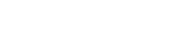# math.atan2

`FLOATmath.atan2FLOATyFLOATx`

Available inall subroutines.

Computes the principal value of the arc tangent of `y`/`x`, using the signs of both arguments to determine the quadrant of the return value.

## Parameters

• `y` - Floating point value.
• `x` - Floating point value.

## Return Value

Upon successful completion, this function returns the arc tangent of `y`/`x` in the range -`math.PI` to `math.PI` radians inclusive.

If `y` is ±0 and `x` is < 0, ±`math.PI` will be returned.

If `y` is ±0 and `x` is > 0, ±0 will be returned.

If `y` is < 0 and `x` is ±0, -`math.PI_2` will be returned.

If `y` is > 0 and `x` is ±0, `math.PI_2` will be returned.

If `x` is 0, a pole error will not occur.

If either `x` or `y` is `math.NAN`, a NaN will be returned.

If `y` is ±0 and `x` is +0, ±0 will be returned.

For finite values of ±`y` > 0, if `x` is `math.NEG_INFINITY`, ±`math.PI` will be returned.

For finite values of ±`y` > 0, if `x` is `math.POS_INFINITY`, ±0 will be returned.

For finite values of `x`, if `y` is `math.POS_INFINITY` or `math.NEG_INFINITY`, ±`math.PI_2` will be returned.

If `y` is `math.POS_INFINITY` or `math.NEG_INFINITY` and `x` is `math.NEG_INFINITY`, ±(3*math.PI_4) will be returned.

If `y` is `math.POS_INFINITY` or `math.NEG_INFINITY` and `x` is `math.POS_INFINITY`, ±math.PI_4 will be returned.

If both arguments are 0, a domain error will not occur.

If the result would cause an underflow, a range error occurs and `math.atan2()` will return `y`/`x`.

## Example

``````declare local var.fo FLOAT;
set var.fo = math.atan2(7, -0);``````

BETA